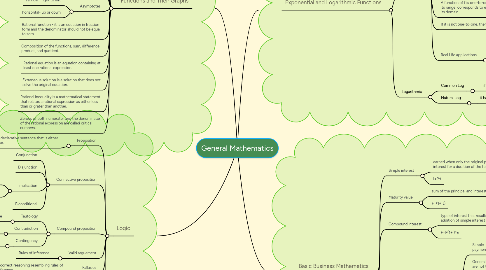# General Mathematics

Get Started. It's FreeGeneral Mathematics## 1. Functions and Their Graphs

### 1.9. Asymptotes

1.9.1. vertical - right to left

1.9.2. horizontal- up or down

## 2. Logic

### 2.1. Proposition

2.1.1. Complete declarative sentence that is either true or false.

### 2.2. Connective proposition

2.2.1. Conjunction

2.2.2. Disjunction

2.2.3. Implication

2.2.3.1. Hypothesis and Conclusion

2.2.3.2. logically equivalent to its contrapositive

2.2.4. Biconditional

### 2.3. Compound proposition

2.3.1. Tautology

2.3.1.1. always true

2.3.2.1. Always false

2.3.3. Contingency

### 2.4. Valid arguement

2.4.1. Rules of inference

### 2.5. Fallasies

2.5.1. incorrect reasoning resembling rules of inference.

### 2.6. Method of proofs

2.6.1. Direct

2.6.2. Indirect

2.6.3. Existence

2.6.4. Nonexistence

## 3. Exponential and Logarithmic Functions

### 3.1. Exponential

3.1.1. If f is increasing or decreasing throughout domain, then f is one-to-one

3.1.2. if each horizontal line intersects the graph in at most one point, then the function if one-to-one.

3.1.3. Laws of exponent

3.1.3.1. (a^x)(a^y)=a^x+y

3.1.3.2. (a^x)^Y=a^xy

3.1.3.3. (ab)^x=a^x b^x

3.1.3.4. (a/b)^x= (a^x)/(b^x)

3.1.3.5. (a^x)/(a^y)=a^x-y

3.1.4. A function of f is one-to-one if every number in its range  corresponds to exactly one number i its domain.

3.1.5. If it is not one-to-one, then their is no inverse.

3.1.6. Real Life applications

3.1.6.1. Half-life decay model

3.1.6.2. Compound interest

3.1.6.3. Doubling time growth

3.1.6.4. Population growth

### 3.2. Logarithmic

3.2.1. Common Log

3.2.1.1. if there is no base, the base will automatically 10

3.2.2. Natural Log

3.2.2.1. it has a base of e written as lnx

### 4.1. Simple interest

4.1.1. earned when only the original principal earns interest for a duration of the term.

4.1.2. I=Prt

### 4.2. Maturity value

4.2.1. sum of the principal and interest rate.

4.2.2. F=(1+rt)

### 4.3. Compound interest

4.3.1. type of interest that results from the periodic addition of simple interest to principal.

4.3.2. F=P(1+i)^n

### 4.4. Annuity -  sequence of equal payment

4.4.1. Simple - interest period is the same as the payment period.

4.4.2. General - interest period and payment period are not the same.

4.4.3. - the payments are made are made at the end of each interest period.

4.4.4. Deffered - the first payment is not made at the end of the first interest period but later.

### 4.5. Stocks

4.5.1. shares of ownership in a company

### 4.6. Bonds

4.6.1. is a loan.

4.6.2. for corporations to raised the necessary funds.

### 4.7. Amortization

4.7.1. dept-repayment scheme wherein the original amount borrowed is repaid by making equal payments periodically.

### 4.8. Outstanding principal

4.8.1. dept that are still unpaid.